# Confidence and Prediction Bands Methods for Nonlinear Models

#### 2022-02-14

Preliminaries: first, we need to load required libraries.

library(ggplot2)
library(nlraa)
library(nlme)
library(mgcv)
## This is mgcv 1.8-34. For overview type 'help("mgcv-package")'.

# Motivation

Why do we need anything other than just the fitted line in regression? The reality is that point estimates hide the uncertainty in our estimates and some form of intervals are crucial for communicating this uncertainty in a visual way. Error bars, confidence and prediction bands can be a convenient and intuitive way of communicating this uncertainty. Unfortunately, their strict statistical interpretation in the frequentist framework might not align with what a reader expects, but I believe that representing uncertainty is one of the main reasons why we go through the troulble of doing statistics. I will next present background on confidence and prediciton bands for linear regression. In part, I’m writing this document for users of this package who will need to know whether any of the different methods are suitable for their applications. There is no one single method for computing this in nonlinear (mixed) models, so having an understanding of the pros and cons can help make decision about which one to use or whether what this package has to offer is appropriate for their application.

## Confidence and Prediciton Bands in Regression

### Linear Example

To illustrate fitting and obtaining confidence and prediction bands in linear regression I will use the Oats data in the nlme package.

data(Oats, package = "nlme")
## A subset for simplicity
Oats.I <- subset(Oats, subset = Block == "I")
plot(Oats.I)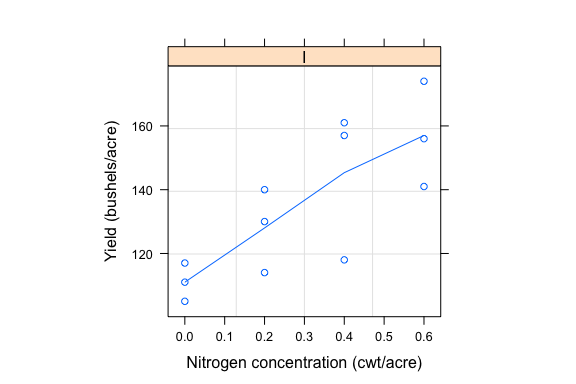Fitting a linear model

fm1 <- lm(yield ~ nitro, data = Oats.I)
fm1.prd <- predict(fm1, interval = "conf")
Oats.IA <- cbind(Oats.I, fm1.prd)
## Make a plot
ggplot(data = Oats.IA, aes(x = nitro, y = yield)) +
geom_point() +
geom_line(aes(y = fit)) +
geom_ribbon(aes(ymin = lwr, ymax = upr), fill = "purple", alpha = 0.4)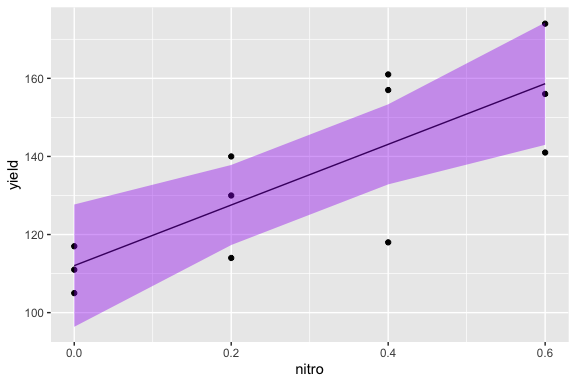The interpretation of these confidence bands is not always intuitive (Krzywinski and Altman, 2013). In the frequentist framework we need to imagine performing this experiment many times and that there is some “true” relationship between nitro and yield. These bands that we create will contain the “true” relationship 95% of the time. However, we do not know whether this particular confidence band contains (or not) the “true” relationship. In more practical terms, displaying these confidence bands along with the relationship is an intuitive way of conveying uncertainty about this relationship. And, in this case, it is clear that this is a result of the very low sample size.

It is also possible to create the same plot using ‘geom_smooth’ in ggplot and the bands are nearly identical (geom_smooth computes predictions at more than just the four points in nitro).

ggplot(data = Oats.IA, aes(x = nitro, y = yield)) +
geom_point() +
geom_line(aes(y = fit)) +
geom_smooth(method = "lm")
## geom_smooth() using formula 'y ~ x'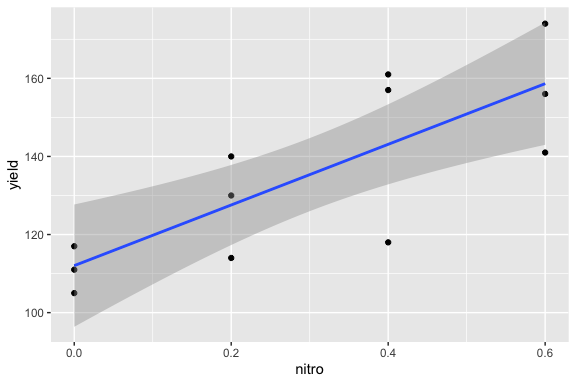Prediction bands (see ?predict.lm) in linear models are a statement about an observation instead of the mean relationship. In the help file it states that these are bands about “future” observations not included in the sample. (Note that these are not always relevant to the specific question being asked.) There are also other kind of intervals such as tolerance intervals (Altman and Krzywinski, 2018).

fm1.prd.int <- predict(fm1, interval = "pred")
## Warning in predict.lm(fm1, interval = "pred"): predictions on current data refer to _future_ responses
Oats.IAP <- cbind(Oats.I, fm1.prd.int)
## Make a plot
ggplot(data = Oats.IAP, aes(x = nitro, y = yield)) +
geom_ribbon(aes(ymin = lwr, ymax = upr), fill = "deepskyblue4", alpha = 0.2) +
geom_ribbon(aes(ymin = Oats.IA$lwr, ymax = Oats.IA$upr), fill = "deepskyblue", alpha = 0.6) +
geom_line(aes(y = fit), color = "white", size = 1.5) +
geom_point() +
ggtitle("Regression Line, 95% Confidence and Prediction Bands")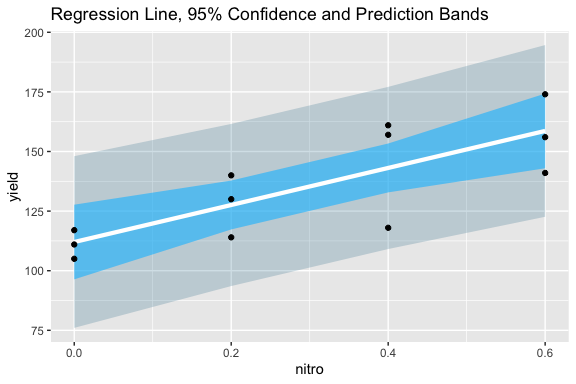It would also be possible to derive these confidence and prediction bands using bootstrapping. This involves resampling the data and computing the fitted values many times. The interval can then be computed from the percentiles from the obtained samples. Is there anything we can learn from doing this?

fm1.boot <- boot_lm(fm1, fitted)
fm1.boot.prd <- summary_simulate(t(fm1.boot$t)) Oats.IAB <- cbind(Oats.I, fm1.boot.prd) ## Make a plot ggplot(data = Oats.IAB, aes(x = nitro, y = yield)) + geom_ribbon(aes(ymin = Q2.5, ymax = Q97.5), fill = "deepskyblue", alpha = 0.6) + geom_line(aes(y = Estimate), color = "white", size = 1.5) + geom_point() + ggtitle("95% Bootstrapped Confidence Bands")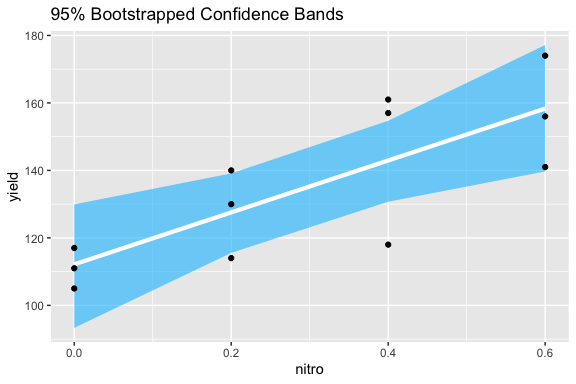It would seem that bootstrapping is unnecessary in linear models when the assumptions are met. An exception can occur if we are interested in a nonlinear combination of parameters from the model. In this case we have two options. First, we could could re-frame the linear model as a nonlinear one. Second, we could use bootstrap to make inferences about this nonlinear function of the linear parameters from the model. The next examples are about nonlinear models. ### Nonlinear Example: Loblolly For a nonlinear example we can use the Loblolly dataset. Lob <- subset(Loblolly, Seed %in% c("301", "303", "305", "307", "309")) fnlm1 <- nls(height ~ SSasymp(age, Asym, R0, lrc), data = Lob) ## Plot of observed and fitted ggplot(Lob, aes(x = age, y = height)) + geom_point() + geom_line(aes(y = fitted(fnlm1)))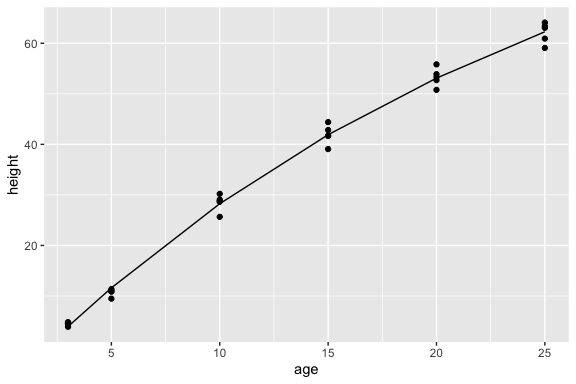The function predict.nls has arguments for the standard error and for intervals but these are currently ignored because (it is considered) that there is no robust method for obtaining these analytically. This to me feels a bit like a Zen teaching from R in which we are supposed to meditate for a long time about what does it mean to not have these values available. So what follows are my thoughts on the matter. There are some discussions in the literature. For example, in Bates and Watts (2007) confidence bands are briefly mentioned (page 58 section 2.3.2). In Venables and Ripley (2000, 2002) bootstrapping is used to approach nonlinear models. This methods is illustrated in more depth in Fox and Weisberg as discussed in the Bootstrapping vignette. An important reason for why bootstrapping is needed is that in nonlinear regression the distribution of the parameter estimates can deviate substantially from a multivariate normal. However, there are a few important points in my opinion: 1. Nonlinear models which have parameters which do present empirical distributions which are close to normal have better statistical properties in general. If this is not the case, it is usually possible to reparameterize them so they are approximately normal. In my experience, nonlinear models with empirical parameter distributions which are far from spherical are harder to fit and less reliable in many ways. This is worse as the model gets more complicated or when we use Bayesian methods. 2. To clarify, it is ok if the parameter estimates distributions deviate from normal to some extent and/or if they are correlated. It is the degree of these two things. If they strongly deviate from normality, this can represent an issue and if the correlation is very high, it might point to an over-parameterized model (but not always!) 3. If we are not comfortable with the normality assumption of the distribution of the parameter estimates in our nonlinear models, then, I would think, that the t-table produced by summary should also be only approximate and not entirely to be trusted. (Let me know if this is not correct.) 4. Therefore, if we have well-behaved nonlinear models then the bootstrap approach will be close to a simpler Monte Carlo approach in which we sample from the distribution of our parameter estimates. (There is an example of this for GAMs in Simon Woods book page 343). 5. An additional consideration is whether there is substantial error in the predictor variables (x). If there is, then case bootstrapping might be preferred compared to the model-based (or residual bootstrap). 6. Bootstrapping, does not lead (in an obvious way) to the estimation of prediction intervals in nonlinear models. Generating them by simulating data from each bootstrap sample is possible, but I’m not sure if it has been demonstrated that this indeed works well. 7. It might be informative to compare the variance-covariance matrix of the parameter estimates in a nonlinear model to the empirical variance-covariance matrix from the bootstrap distribution. Strong deviations are probably an indication of a model that will not be well-behaved. After these observations, we can continue with the Loblolly example. Lob.bt.pe <- boot_nls(fnlm1) ## This takes a few seconds (~7s) pairs(Lob.bt.pe$t, labels = c("Asym", "R0", "lrc"))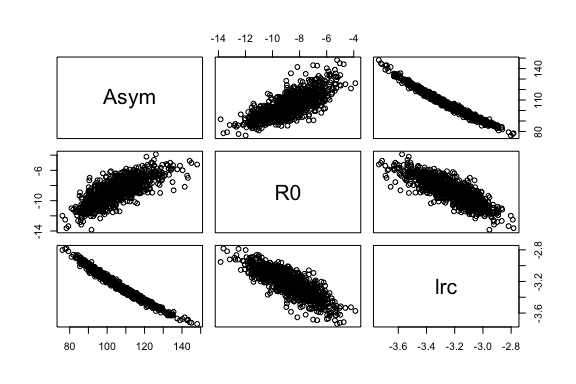print(cov2cor(var(Lob.bt.pe$t)), digits = 2) ## Correlation matrix ## [,1] [,2] [,3] ## [1,] 1.00 0.76 -0.99 ## [2,] 0.76 1.00 -0.82 ## [3,] -0.99 -0.82 1.00 These distributions deviate somewhat from a multivariate normal. (A formal test would reject the hypothesis that the data is MVN - not shown.) Here I propose to compute confidence bands for these data using the following methods: 1. A polynomial linear model 2. A nonlinear model and the Delta Method 3. A nonlinear model and bootstrap 4. A nonlinear model and Monte Carlo 5. A GAM model ## Linear model fm1.Lob <- lm(height ~ poly(age, 3), data = Lob) fm1.Lob.prd <- predict(fm1.Lob, interval = "conf") fm1.Lob.dat <- data.frame(method = "lm-poly(3)", Lob, fm1.Lob.prd) ## Nonlinear model + Delta Method fm2.Lob <- nls(height ~ SSasymp(age, Asym, R0, lrc), data = Lob) fm2.Lob.dm <- predict2_nls(fm2.Lob, interval = "conf") fm2.Lob.dm.dat <- data.frame(method = "Delta-Method", Lob, fit = fm2.Lob.dm[,1], lwr = fm2.Lob.dm[,3], upr = fm2.Lob.dm[,4]) ## Nonlinear model + bootstrap fm2.Lob <- nls(height ~ SSasymp(age, Asym, R0, lrc), data = Lob) fm2.Lob.bt <- boot_nls(fm2.Lob, fitted) ## This takes about 7s fm2.Lob.prd <- summary_simulate(t(fm2.Lob.bt$t))
fm2.Lob.bt.dat <- data.frame(method = "nls-bootstrap", Lob,
fit = fm2.Lob.prd[,1],
lwr = fm2.Lob.prd[,3],
upr = fm2.Lob.prd[,4])
## Nonlinear model + Monte Carlo
fm2.Lob.MC <- predict_nls(fm2.Lob, interval = "conf")
fm2.Lob.MC.dat <- data.frame(method = "nls-Monte-Carlo", Lob,
fit = fm2.Lob.MC[,1],
lwr = fm2.Lob.MC[,3],
upr = fm2.Lob.MC[,4])
## GAM
fm3.Lob <- gam(height ~ s(age, k = 3), data = Lob)
fm3.Lob.prd <- predict(fm3.Lob, se.fit = TRUE)
fm3.Lob.GAM.dat <- data.frame(method = "GAM", Lob,
fit = fm3.Lob.prd$fit, lwr = fm3.Lob.prd$fit - 2 * fm3.Lob.prd$se.fit, upr = fm3.Lob.prd$fit + 2 * fm3.Lob.prd$se.fit) prd.all <- rbind(fm1.Lob.dat, fm2.Lob.dm.dat, fm2.Lob.bt.dat, fm2.Lob.MC.dat, fm3.Lob.GAM.dat) ### Finally plot ggplot(data = prd.all, aes(x = age, y = height)) + facet_wrap(facets = "method") + geom_line(aes(y = fit)) + geom_point() + geom_ribbon(aes(ymin = lwr, ymax = upr), fill = "purple", alpha = 0.5)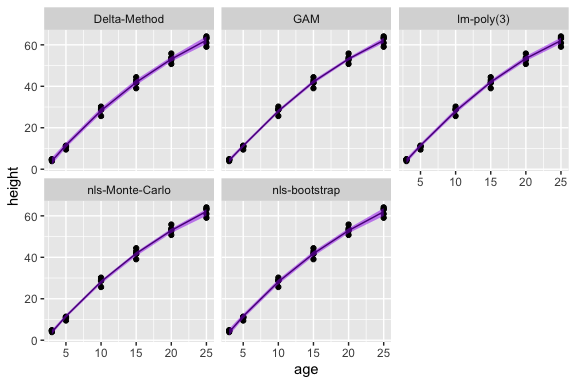The differences among the methods are minor and in practical terms should not result in different decisions. Apparently, the bootstrap method is slightly wider than the other three, but by a small margin. If we are willing to accept that these methods are approximately similar even under conditions which deviate from the ideal, we might be willing to extend these methods to more complex models. ### Nonlinear Example: Puromycin The Puromycin dataset was used in the Book by Bates and Watts and confidence bands are briefly described in pages 58-59. They report a 95% confidence band at x = 0.4 of [171.6, 195]. Their method is known as the Delta method and it is implemented in function predict2_nls. (Clearly, I thought of implementing this method at a later time. The issue is that I was trying to find a method that would extend to more complex models: nlme). PurTrt <- Puromycin[ Puromycin$state == "treated", ]
fm1.P <- nls(rate ~ SSmicmen(conc, Vm, K), data = PurTrt)
## Confidence bands using the Delta method
fm1.P.dm <- predict2_nls(fm1.P, interval = "conf")
## Reproducing book results
round(predict2_nls(fm1.P, interval = "conf", newdata = data.frame(conc = 0.4)), 2)
##   Estimate Est.Error   Q2.5  Q97.5
## 1    183.3      4.07 171.64 194.96
PurTrtA.dm <- cbind(PurTrt, fm1.P.dm)
ggplot(data = PurTrtA.dm, aes(x = conc, y = rate)) +
geom_point() +
geom_line(aes(y = fitted(fm1.P))) +
geom_ribbon(aes(ymin = Q2.5, ymax = Q97.5), fill = "purple", alpha = 0.2) +
ggtitle("95% Delta Method Confidence Bands")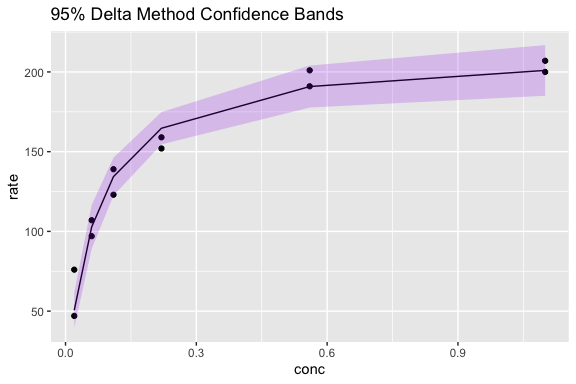## Confidence bands using the bootstrap method
fm1.P.bt <- boot_nls(fm1.P) ## this takes about 5 seconds
pairs(fm1.P.bt$t, labels = c("Vm", "K"))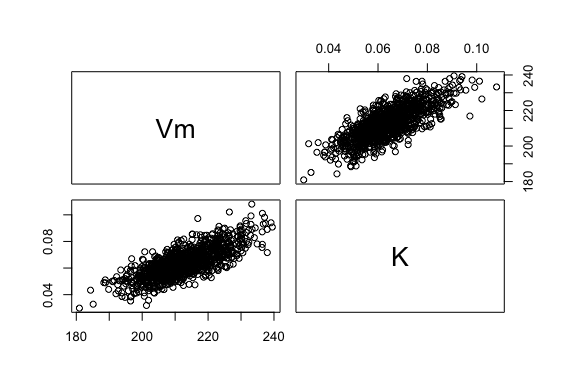## Bootstrapped confidence bands fm1.P.bt.ft <- boot_nls(fm1.P, fitted) ## This takes about 5s fm1.P.bt.ft.prd <- summary_simulate(t(fm1.P.bt.ft$t))
PurTrtA <- cbind(PurTrt, fm1.P.bt.ft.prd)
## Plot data and bands
ggplot(data = PurTrtA, aes(x = conc, y = rate)) +
geom_point() +
geom_line(aes(y = fitted(fm1.P))) +
geom_ribbon(aes(ymin = Q2.5, ymax = Q97.5), fill = "purple", alpha = 0.2) +
ggtitle("95% Bootstrap Confidence Bands")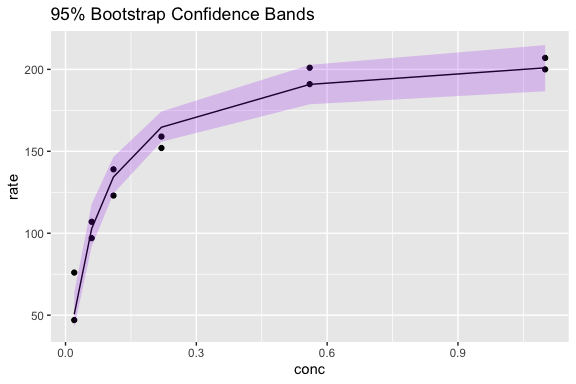## Predictions at 0.4
prd_fun <- function(x) predict(x, newdata = data.frame(conc = 0.4))
prd_fun(fm1.P) ## It also provides the gradient
##  183.3001
##             Vm         K
## [1,] 0.8618438 -394.9402
fm1.P.at.x.0.4 <- boot_nls(fm1.P, prd_fun) ## This takes about 6s
boot::boot.ci(fm1.P.at.x.0.4, type = "perc") 
## BOOTSTRAP CONFIDENCE INTERVAL CALCULATIONS
## Based on 999 bootstrap replicates
##
## CALL :
## boot::boot.ci(boot.out = fm1.P.at.x.0.4, type = "perc")
##
## Intervals :
## Level     Percentile
## 95%   (172.6, 194.8 )
## Calculations and Intervals on Original Scale

The boostrap estimate is similar to the confidence band from the book. How does the Monte Carlo approach compare?

(prd.at.x.0.4 <- predict_nls(fm1.P, newdata = data.frame(conc = 0.4)))
##  183.3001

So, it is closer to the bootstrap than the Delta method method.

ndat <- data.frame(conc = seq(0, 1.3, length.out = 50))
Pprd <- predict_nls(fm1.P, interval = "conf",
newdata = ndat)
Pprdd <- data.frame(ndat, Pprd)
ggplot() +
geom_point(data = PurTrt, aes(x = conc, y = rate)) +
geom_line(data = Pprdd, aes(x = conc, y = Estimate)) +
geom_ribbon(data = Pprdd, aes(x = conc, ymin = Q2.5, ymax = Q97.5),
fill = "purple", alpha = 0.4) +
ggtitle("Monte Carlo 95% Confidence Bands")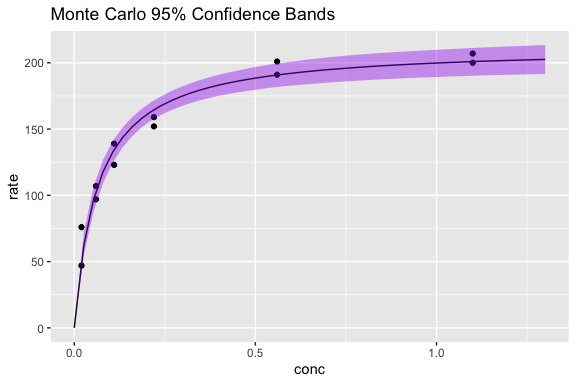In this case, the Delta Method, Bootstrap and Monte Carlo are very similar and it is unlikey that their difference would lead to different decisions in practical terms.

## Nonlinear Example: Maize Leaf Extension Rate

This is a more pathological example in which the model is harder to fit and it presents some challenges.

data(maizeleafext)
## Display the data
fmm1 <- nls(rate ~ SStemp3(temp, t.m, t.l, t.h), data = maizeleafext)
ggplot(data = maizeleafext, aes(x = temp, y = rate)) +
geom_point() + geom_line(aes(y = fitted(fmm1)))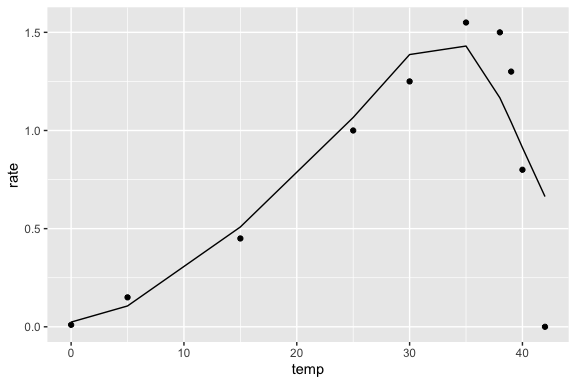## The model seems slightly inadequate for these data
fmm1.dm <- predict2_nls(fmm1, interval = "conf")
mlf <- cbind(maizeleafext, fmm1.dm)
## The confidence bands are fairly wide
ggplot(data = mlf, aes(x = temp, y = rate)) +
geom_point() + geom_line(aes(y = fitted(fmm1))) +
geom_ribbon(aes(ymin = Q2.5, ymax = Q97.5),
fill = "purple", alpha = 0.3)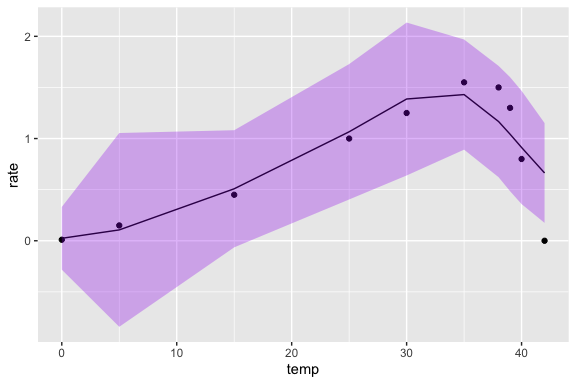## What about bootstrap?
fmm1.bt <- boot_nls(fmm1) ## This takes about 5s
## Notice that the model does not converge in many instances
pairs(fmm1.bt$t, labels = c("t.m", "t.l", "t.h"))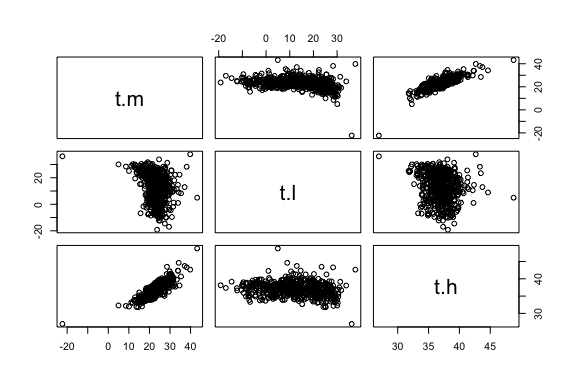## Nonlinear Example: Theoph There are not too many attempts in the literature to investigate methods and performance of confidence bands in nonlinear mixed models. One paper which explores this is by Gsteiger et al. (2011) “Simultaneous confidence bands for nonlinear regression models with application to population pharmacokinetic analyses”. In their analysis they explore one method based on Schwartz inequality for an approximate analytical approach and a Monte Carlo method. They conclude that the analytical approach tends to be conservative (i.e. overcoverage) and the Monte Carlo approach tends to be liberal (i.e. undercoverage). Their analytical approach, however, requires the calculation of the gradient and in their R code they use the fact that the SSfol function has analytical derivatives of the function with respect to the parameters. When these are not available, they would need to be computed numerically and this has its own challenges. Their Monte Carlo approach differs from the one I use, but I have not looked into this in detail yet. However, below I present confidence bands based on my approach for the dataset they use in that publication. (I also search within papers which cited the Gsteiger et al. publication and did not find any new developments). data(Theoph) fmL.Theoph <- nlsList(conc ~ SSfol(Dose, Time, lKe, lKa, lCl), data = Theoph) fm0.Theoph <- nlme(fmL.Theoph, random = pdDiag(lKa + lCl ~ 1)) ## The Dose is different for each subject however... ndat <- data.frame(Dose = median(Theoph$Dose), Time = seq(0, 25, by = 0.1))
fm0.Theoph.prd <- predict_nlme(fm0.Theoph, newdata = ndat, interval = "conf")
fm0.Theoph.prd.dat <- cbind(ndat, fm0.Theoph.prd)
## plot data
ggplot() +
geom_point(data = Theoph, aes(x = Time, y = conc)) +
geom_line(data = fm0.Theoph.prd.dat, aes(x = Time, y = Estimate)) +
geom_ribbon(data = fm0.Theoph.prd.dat,
aes(x = Time, ymin = Q2.5, ymax = Q97.5), fill = "purple", alpha = 0.3) +
xlab("Time [h]") + ylab("Theophylline concentration [mg/L]") +
ggtitle("95% confidence bands")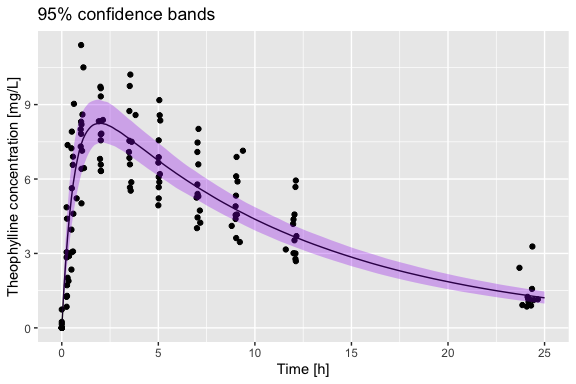I’m somewhat confused by their statement that it is not possible to compute prediction bands. Their statement is “Also, having considered confidence bands, it is natural to wonder whether one could construct simultaneous prediction bands. However, this is not possible, as shown by Donnelly (2003)”. A Bayesian approach will certainly be able to give you an answer to this question, but I’m not sure how “good” that answer is expected to be… but this is very different from “impossible”. I think that the issue is that it might not be clear what is meant by prediction in this case. Is it for an observation within the regression of a specific subject? Or is it a prediction band for the mean function of any subject? I have implemented the ability to sample new random effects (using psim = 3), which can be used to compute prediction bands for a new subject.

### Nonlinear Example: Prediction bands for an observation within a subject

To compute the uncertainty for observations within the regression for a given subject, this is how we can approach it. These bands are a statement about the uncertainty of observations around the regression line for each subject.

fm0.Theoph.prd.bnd <- predict_nlme(fm0.Theoph, plevel = 1, interval = "pred")
fm0.Theoph.prd.bnd.dat <- cbind(Theoph, fm0.Theoph.prd.bnd)
## Plot it
ggplot(data = fm0.Theoph.prd.bnd.dat, aes(x = Time, y = conc)) +
facet_wrap(~Subject) +
geom_point() +
geom_line(aes(x = Time, y = Estimate)) +
geom_ribbon(data = fm0.Theoph.prd.bnd.dat,
aes(x = Time, ymin = Q2.5, ymax = Q97.5), fill = "purple", alpha = 0.3) +
xlab("Time [h]") + ylab("Theophylline concentration [mg/L]") +
ggtitle("95% prediction bands (within subjects)")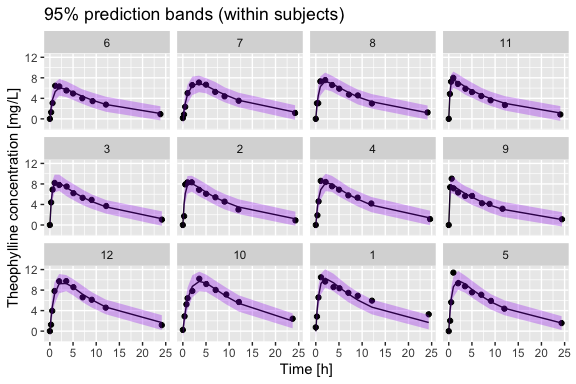### Nonlinear Example: Prediction bands for an mean function (new subject)

This question might be slightly harder conceptually. If we were to sample a new subject, we would want a regression band that will likely contain it at a specified probability level. Of course, the probability level should work in repeated sampling, but not for any given specific subject. First, let’s look at the variability between the existing subjects.

ndat <- expand.grid(Dose = median(Theoph$Dose), Time = 0:25, Subject = unique(Theoph$Subject))
ndat$Estimate <- predict(fm0.Theoph, newdata = ndat, level = 1) fm0.Theoph.simA <- ndat ## Plot the simulations ggplot() + geom_point(data = Theoph, aes(x = Time, y = conc)) + geom_line(data = fm0.Theoph.simA, aes(x = Time, y = Estimate, group = Subject), color = "gray") + geom_ribbon()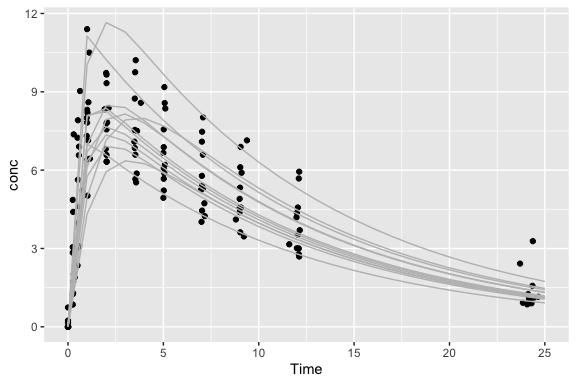To get prediciton bands at this level I will use bootstrapping. NOTE: I’m not running the code in this vignette because it takes a long time and it produces a large object, but I encourage the interested readers to run this. pred_band_BS <- function(x) predict(x, newdata = ndat, level = 1) fm0.Theoph.bt <- boot_nlme(fm0.Theoph, pred_band_BS) ## This takes a bit over a minute fm0.Theoph.bt.ss <- cbind(ndat[,-4], summary_simulate(t(na.omit(fm0.Theoph.bt$t))))
fm0.Theoph.bt.ss.A <- aggregate(cbind(Estimate, Est.Error, Q2.5, Q97.5) ~ Time,
data = fm0.Theoph.bt.ss, FUN = mean)
## plot data
ggplot() +
geom_point(data = Theoph, aes(x = Time, y = conc)) +
geom_line(data = fm0.Theoph.bt.ss.A, aes(x = Time, y = Estimate)) +
geom_ribbon(data = fm0.Theoph.bt.ss.A, aes(x = Time, ymin = Q2.5, ymax = Q97.5), fill = "purple", alpha = 0.3) +
xlab("Time [h]") + ylab("Theophylline concentration [mg/L]") +
ggtitle("95% prediction bands (between subjects)")

In the previous calculation of prediction bands I have fixed the Dose at the median value, this is part of the reason why the uncertainty seems lower than it should be. The other reason is that I am not taking into account the uncertainty from the random effects. (This is possible for lme or nlme objects: see psim = 3 in the simulate_ functions or the new-prediction option in the predict_ functions).